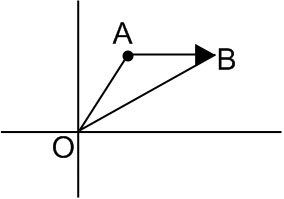## Filters

Q

The value of , for which the points with position  vectors   respectively are the vertices of a right angled triangle at are

• Option 1)

2 and 1

• Option 2)

–2 and –1

• Option 3)

–2 and 1

• Option 4)

2 and –1

95 Views

As we have learned

Scalar Product of two vectors -

- wherein

is the angle between the vectors

Position Vector -

If  and  are the position of vectors of two points A and B then

- wherein( a-2 )( a-1 )= 0

a = 1,2

Option 1)

2 and 1

Option 2)

–2 and –1

Option 3)

–2 and 1

Option 4)

2 and –1

Exams
Articles
Questions